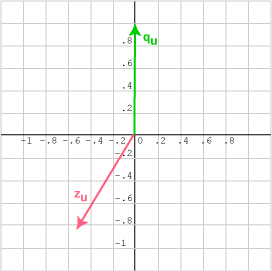Now, cos θ = -0.866. So θ = arc cos -0.866 = 150°.

# Angles Greater than 90```

```

qu  =  (0.0, 1)T and zu   =  (-0.5, -0.866)T

The angle between the two vectors is greater than 90°, so the cosine is negative.

### QUESTION 11:

In the diagram above, mentally draw the other vector that has a dot product of -0.866 with vector qu.